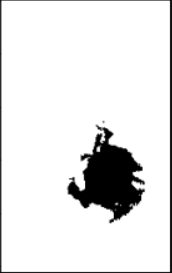# Problem in plotting the masks

Hello everyoneI am working on the segmentation problem and images are grayscale but I stacked them along the depth which made each channel to be a 4 channel image and I also did the one hot encoding for my mask there were 4 labels in total one of them was the background (I also want to know how to exclude that altogether). My average IoU score was 0.86 keeping the threshold to be 0.9 but when I finally want to generate the mask this is the output I am getting.Any idea what might be wrong?

Here’s my code:

``````%matplotlib inline
import os
import warnings
warnings.filterwarnings("ignore", category=RuntimeWarning)
warnings.filterwarnings("ignore", category=UserWarning)

import random

import pandas as pd
import numpy as np

import nibabel as nib

import nibabel as nib
import tarfile

import tensorflow as tf

import torch
from torch.nn.functional import one_hot
from torch import nn, optim
from torch.optim.lr_scheduler import LambdaLR

import segmentation_models_pytorch as smp

import albumentations as A
from albumentations.pytorch import ToTensorV2

import cv2

from tqdm.auto import tqdm

import matplotlib.pyplot as plt
import seaborn as sns

if torch.cuda.is_available():
device = "cuda:0"
torch.cuda.empty_cache()
else:
device = "cpu"

SEED = 42

np.random.seed(SEED)
torch.manual_seed(SEED)

torch.cuda.empty_cache()
device = torch.device(device)

class datagen(Dataset):
def __init__(self, IDs, tfms):
super(datagen, self).__init__()
self.IDs = IDs
self.tfms = tfms

def __len__(self):
return len(self.IDs)

def __getitem__(self, idx):
ID = self.IDs[idx]

y[y == 4] = 3

images = []

for i in range(len(x1)):
image = np.dstack((x1[i], x2[i], x3[i], x4[i]))
if np.count_nonzero(image) == 0:
image = tfms(image = image)['image']
else:
image = (image - np.min(image))/(np.max(image) - np.min(image))
image = tfms(image = image)['image']
images.append(image)

class Network(nn.Module):
def __init__(self):
super(Network, self).__init__()
self.model = smp.Unet(encoder_name = 'efficientnet-b7',
encoder_weights = 'imagenet',
in_channels = 4,
classes = 4,
activation = 'sigmoid')
for param in self.model.encoder.parameters():
def forward(self, x):
x = self.model(x)
return x

ids = os.listdir('./data')
random.shuffle(ids)
train_ids = ids[: 1002]
val_ids = ids[1002: ]

tfms = A.PadIfNeeded(min_height = 256, min_width = 160, always_apply = True)

train_set = datagen(train_ids, tfms)
train = DataLoader(train_set, batch_size = 1, shuffle = True)

val_set = datagen(val_ids, tfms)
val = DataLoader(val_set, batch_size = 1, shuffle = True)

torch.cuda.empty_cache()
model = Network().to(device)
criterion = smp.utils.losses.DiceLoss()
metrics = [smp.utils.metrics.IoU(threshold=0.95)]
optimizer = torch.optim.Adam(model.parameters(), lr = 0.005)
epochs = 1

for e in range(epochs):
train_losses = []
train_iou = []
val_losses = []
val_iou = []
for i in range(len(image)):
x, y = torch.tensor(image[i], dtype = torch.float32).reshape(1, 4, 256, 160).to(device), torch.tensor(mask[i], dtype = torch.float32).reshape(1, 4, 256, 160).to(device)
y_pred = model.forward(x)
loss = criterion(y_pred, y)
train_losses.append(loss.item())
iou = metrics.forward(y_pred, y)
train_iou.append(iou)
loss.backward()
optimizer.step()
print(f'Loss: {loss.item():.4f}, IoU: {iou:.4f}', end = '\r')
for i in range(len(image)):
x, y = torch.tensor(image[i], dtype = torch.float32).reshape(1, 4, 256, 160).to(device), torch.tensor(mask[i], dtype = torch.float32).reshape(1, 4, 256, 160).to(device)
y_pred = model.forward(x)
loss = criterion(y_pred, y)
val_losses.append(loss.item())
iou = metrics.forward(y_pred, y)
val_iou.append(iou)
print(f'Loss: {loss.item():.4f}, IoU: {iou:.4f}', end = '\r')

print(f'Epochs: {e + 1}/{3}, Training Loss: {sum(train_losses)/len(train_losses)}, Training IoU: {sum(train_iou)/len(iou)}, Validation Loss: {sum(val_losses)/len(val_losses)}, Validation IoU: {sum(val_iou)/len(val_iou)}')
torch.save(model.state_dict, f'./model_{1}.pth')

y_pred = model.forward(torch.tensor(images.reshape(1, 4, 256, 160), dtype = torch.float32).cuda())
y = masks.reshape(1, 4, 256, 160)
b = criterion(y_pred, y.cuda())
``````

(background, not necessary)The images were 240, 155 initially but I padded them to 256, 160 because it was required by the frameworok to have a image of the dimensions divisible by 32

I don’t know what memory layout `nib` uses, but I would guess it’s channels-last (same as OpenCV)?
If so, you are interleaving the images most likely via:

``````images.reshape(1, 4, 256, 160)
``````

and should change it to a `tensor.permute` or `numpy_array.transpose` if you want to change the dimension order.

1 Like

Thank you Peter, this seems to work.